Download Presentation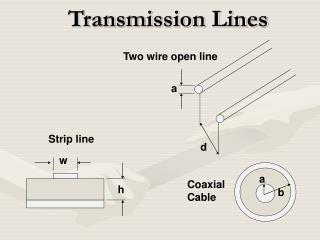Transmission Lines

# Transmission Lines - PowerPoint PPT Presentation

Two wire open line. Transmission Lines. a. Strip line. d. w. a. Coaxial Cable. h. b. Two Wire Open Line. E x = E 0 exp (i[kz -  t]), H y = (E 0 /Z 0 ) exp (i[kz-  t]).I am the owner, or an agent authorized to act on behalf of the owner, of the copyrighted work described.
Download Presentation## Transmission Lines

An Image/Link below is provided (as is) to download presentation

Download Policy: Content on the Website is provided to you AS IS for your information and personal use and may not be sold / licensed / shared on other websites without getting consent from its author.While downloading, if for some reason you are not able to download a presentation, the publisher may have deleted the file from their server.

- - - - - - - - - - - - - - - - - - - - - - - - - - E N D - - - - - - - - - - - - - - - - - - - - - - - - - -
Presentation Transcript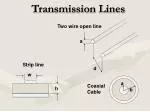Two wire open line

### Transmission Lines

a

Strip line

d

w

a

Coaxial Cable

h

b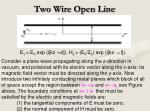Two Wire Open Line

Ex = E0 exp (i[kz -t]), Hy = (E0/Z0) exp (i[kz- t]).

• Consider a plane wave propagating along the z-direction in vacuum, and polarized with its electric vector along the x-axis: its magnetic field vector must be directed along the y-axis. Now introduce two infinitely conducting metal planes which block of all of space except the region between x= +a and x= -a, see Figure above. The boundary conditions at x=  a that must be
• satisfied by the electric and magnetic fields are:
• (1) the tangential components of E must be zero;
• (2) the normal component of H must be zero.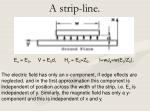A strip-line.

Ex = E0, V = E0d, Hy = E0=Z0, I=wJs=w(E0/Z0).

The electric field has only an x-component, if edge effects are

neglected, and in the first approximation this component is independent of position across the width of the strip, i.e. Ex is independent of y. Similarly, the magnetic field has only a y-component and this is independent of x and y.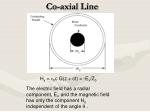Co-axial Line

Hy = 0c G(z + ct) = -Ex/Z0

The electric field has a radial component, Er, and the magnetic field has only the component H

independent of the angle  .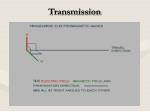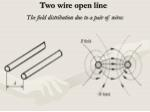Two wire open line

The field distribution due to a pair of wires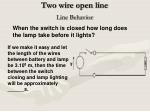Two wire open line

When the switch is closed how long does the lamp take before it lights?

Line Behavior

If we make it easy and let the length of the wires between battery and lamp be 3.108 m, then the time between the switch closing and lamp lighting will be approximately ______s.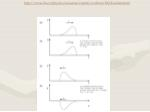http://www.sfu.ca/physics/associate/emeriti/cochran/MAXoutline.htmlhttp://www.sfu.ca/physics/associate/emeriti/cochran/MAXoutline.html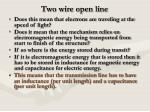Does it mean that the mechanism relies on electromagnetic energy being transported from start to finish of the structure?

If so where is the energy stored during transit?

If it is electromagnetic energy that is stored then it has to be stored in inductance for magnetic energy and capacitance for electric energy.

This means that the transmission line has to have an inductance (per unit length) and a capacitance (per unit length).

Two wire open line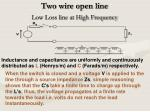Two wire open line

Low Loss line at High Frequency

Inductance and capacitance are uniformly and continuously distributed as L (Henrys/m) and C (Farads/m) respectively.

When the switch is closed and a voltage V is applied to the line through a source impedance Zs, simple reasoning shows that the C's take a finite time to charge up through the L's; thus, the voltage propagates at a finite rate towards the load i.e. volts do not reach the load instantaneously.Transmission in Capacitive Element

Let L and C be distributed inductance

and capacitance per unit length.

In time t, electric flux q = (C ·x)v ( Q = CV) is

produced in time x/u sec , and

i(amps) = q/ t = Cx• v/ t

(capacitive charging or "displacement" current)

i = Cx• v /(x/ u ) = Cvu (I)Transmission in Magnetic Element

In time t, magnetic flux linkages =(L•x)i

(from = LI) ; are produced in time x/u sec

v(volts) = /t = L•x•i/t=Liu (ind Volts)…(II)

Multiplying I and II:- vi =viCLu2

Or u = 1/  LC ms-1velocity of propagation

Dividing I by II:- i/v =vC/iL or v2/i2 =L/C

v/I = L/C……Characteristic Impedance Z0Energy Transmission

v2

L

=

; i2 L = v2C

i2

C

½ Li2 = ½ Cv2

Note:- the equality of Electric and Magnetic field energy in unit length of transmission line.Movement of Energy

Rate of energy input to line due to advance of wavefront, is vi watts.

resistive load, connected directly to voltage source. Rate of energy input, due to thermal dissipation in R, is vi watts (also i2R).Movement of Energy

Rate of energy input = vi watts. Idea of a

'matched line': looks like an infinitely

long line.

Note: if Rg = Z0, maximum power if transferred from the generator to the line.Movement of Energy

As can be seen from the intuitive picture of a transmission line, wave propagation characteristics are dependent on the inductance and capacitance of the line. Thus we need to find expressions for the L and C of typical lines.Inductance in Two wire line

Inductance

Assume r << S

Due to conductor A,

for 1 amp Linkages per metre, axially,

LInductance in Two wire line

Due to conductor B, an identical expression is

Obtained:-Mutual Inductance Between 2 Twin Lines

External field due to 1 amp in line A, at radiusMutual Inductance Between 2 Twin Lines

Flux linkages per metre axially through circuit

(between conductor 1 & 2) is:-

 =

B =Capacitance two wire line

Capacitance of a Twin Line (r << S)

Assign a line charge of 1 C/m to both conductors.

D at x from conductor A is;Capacitance two wire line

and

Similarly for VB(=VA)R, L, C of Two wire line

per unit length

Rdc=

ohm/m

2A2

0

d

loge

loge

L =

H/m

a

0

C =

F/m

loge (d/a)Z0Twin-wire line

Characteristic Impedence

For air, therefore Z0 = 120 loge(b/a), (approx )

0

d

loge

L =

a

0

C =

loge (d/a)